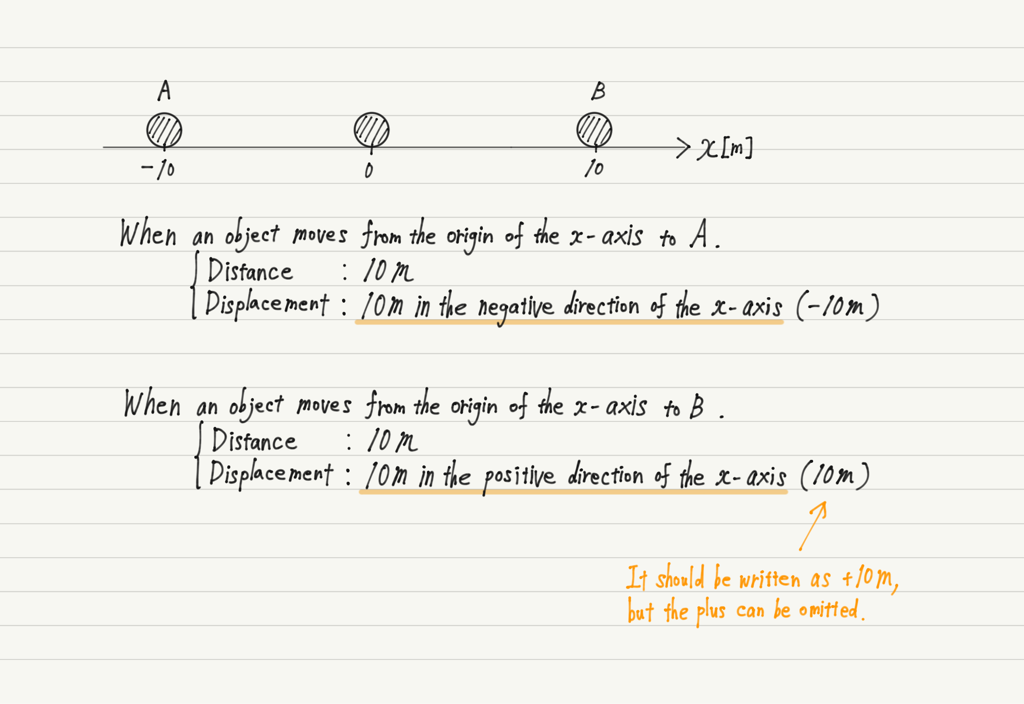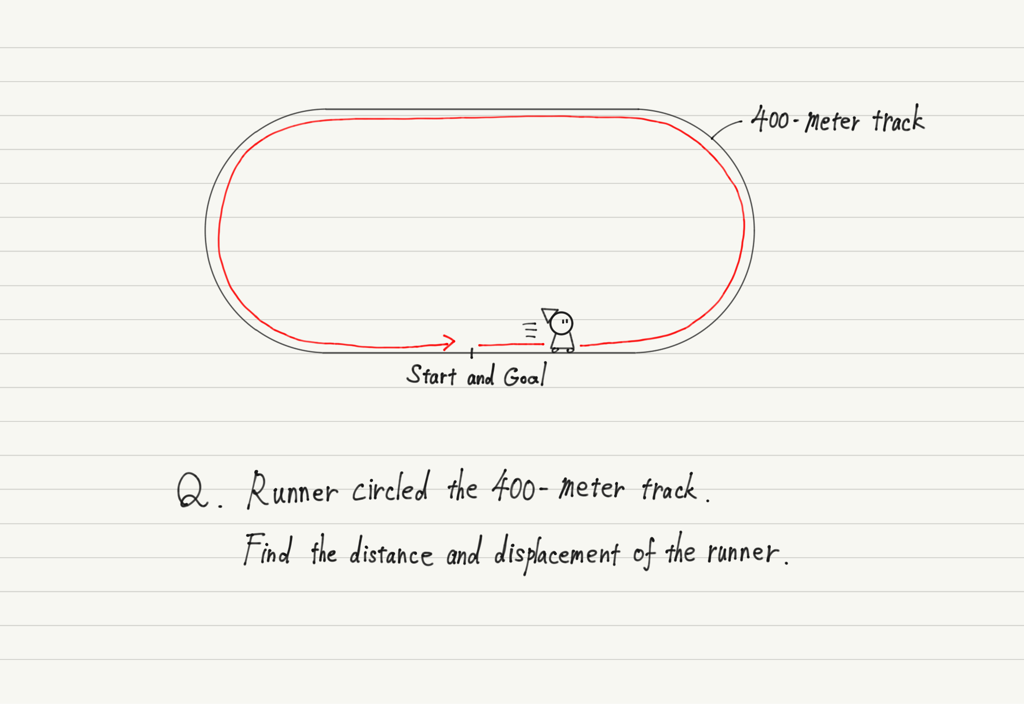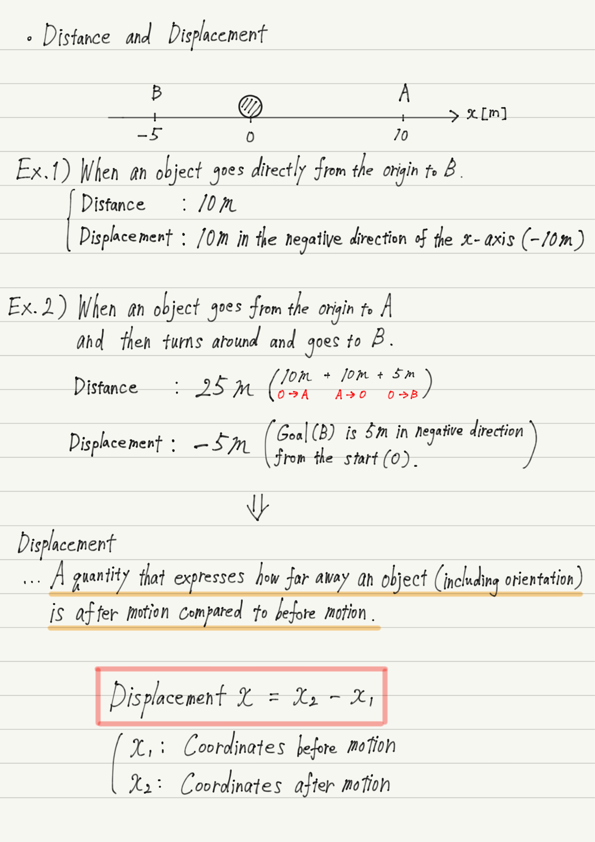# Distance and Displacement

Continuing on from the previous lecture, we will be presenting the theme of the use of different terminology.

The topics covered in this lecture are “Distance” and “Displacement”.
Let’s review the meaning of each term.

## Difference between Distance and Displacement Part 1

Displacement is “a change in the position of an object in motion”.

To be precise, it is “how much the position of an object after motion deviates from its initial position.”

However, I think this explanation does not make the difference between displacement and distance clear.
To clarify the difference, let us look at an example.Distance is simply “how many meters have you moved”, whereas displacement is “the deviation from the initial position”, so it must also include information about which direction the object has shifted!

Two pieces of information are needed, the numerical value and the orientation, just like the relationship between speed and velocity that we discussed in the last lecture!

## Difference between Distance and Displacement Part 2

There are other significant differences between distance and displacement besides the presence or absence of orientation, so let’s look at one last example.What would be the answer to this question?

Now, I would like to explain the question.
The distance is simple. The runner travels one lap around a 400-meter track, so the distance traveled by the runner is 400 m.

On the other hand, what about displacement?

Again, displacement is “how much the position of an object after motion deviates from its initial position.”

The runner has completed one lap of the track and is back at the starting point again. That is, their position after the motion is the same as their initial position.
Therefore, in this case, we conclude that the displacement is zero.

In summary, the answer to the above example is: Distance: 400 m, Displacement: 0 m.

Some may find it disconcerting that “displacement is zero even if you don’t move from the starting point, and displacement is zero after one lap,” but the usefulness of the concept of displacement becomes apparent with a little more study!

## Summary of this lecture## Next Time

In the next lecture, we’ll learn a new concept: Acceleration!

https://www.yukimura-physics.com/entry/dyn-f05

error: Content is protected !!